# Square Unit – Definition with Examples

» Square Unit – Definition with Examples

## What are Square Units?

In geometry, a square unit can be defined as the metric unit used to measure area.

Here, for instance, the area of this rectangle is measured in square units using unit squares.

Some examples of square units in metric units are square meters, square centimeters, and in customary units are square inches, square feet.

##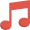Let’s sing!

Let’s measure the area of the big garden pit,
Multiply length- breadth, and write its square units.

##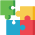Let’s do it!

While solving area word problems, emphasize on how the single unit of length or breadth turns into square units while finding the area.

##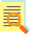Related math vocabulary

AreaShapes

Play Now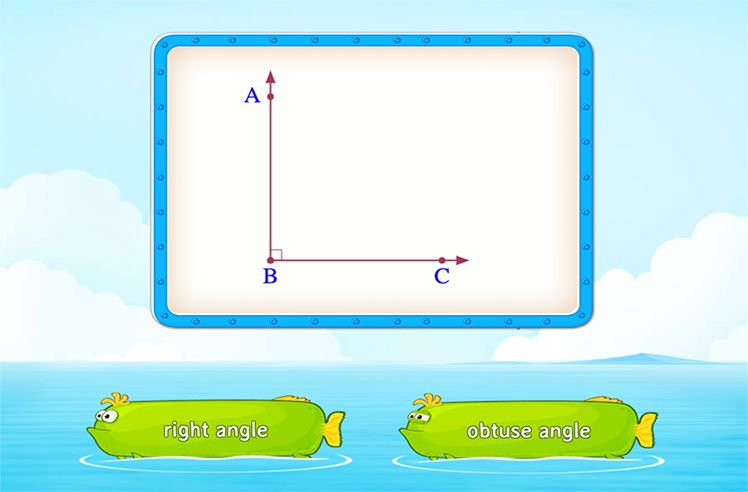Angles

Play Now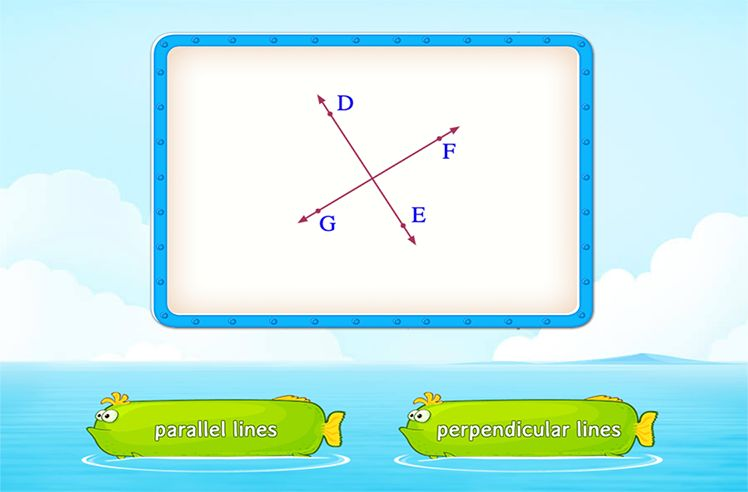Parallel and Perpendicular Lines

Play Now